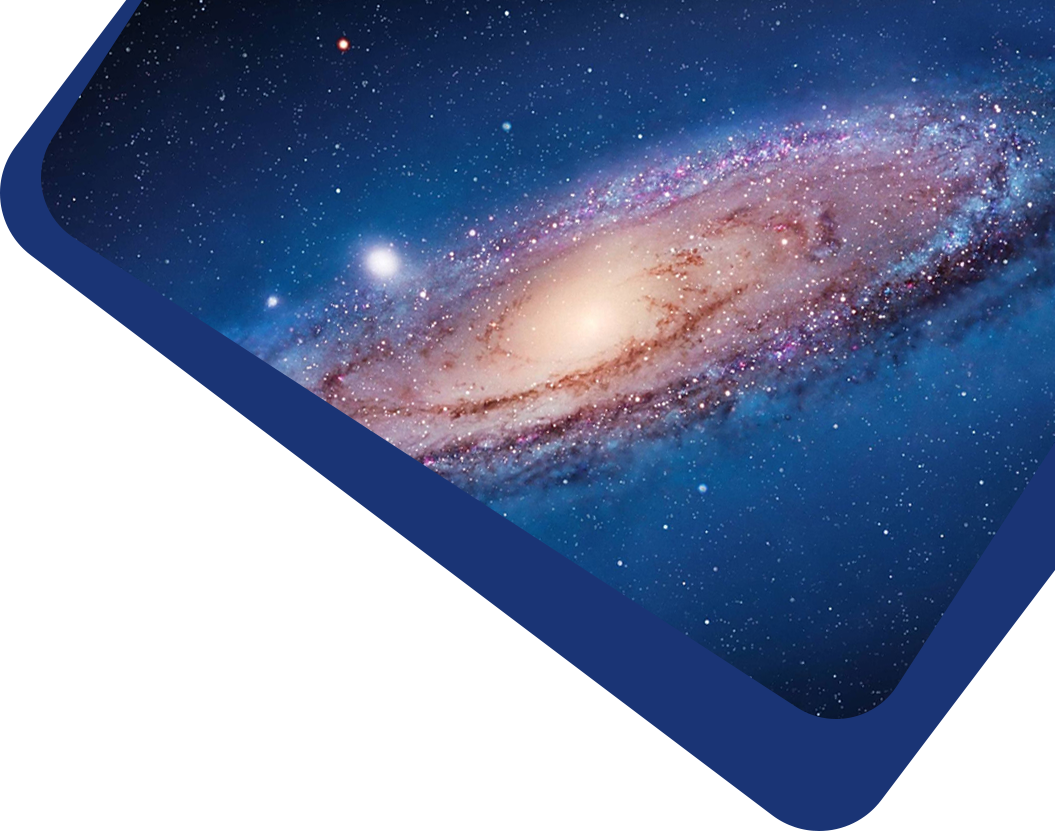Vol 22, No 8

## Correlation between Brightness Variability and Spectral Index Variability for Fermi Blazars

#### Wen-Xin Yang, Hu-Bing Xiao, Hong-Guang Wang, Jiang-He Yang, Zhi-Yuan Pei et al.

Abstract

In this work, the γ-ray photon flux, photon spectral index ($$\alpha_{ph}$$), variability index (log V.I.), and the synchrotron peak frequency (log $$\nu_p$$) are compiled for 851 common blazars from the 3FGL and 4FGL catalogs and Fan et al. to investigate variability properties for Fermi blazars. Our calculations and analyses reach following results: (1) the averaged luminosity, spectral index, and variability index of FSRQs are higher than those of BL Lacs for the whole sample. (2) It is found that the spectral index variation is closely anti-correlated with the luminosity variation implying that the spectrum becomes harder when the source becomes brighter in the γ-ray band. (3) Positive correlations are found between the photon spectral index and both γ-ray luminosity and variability index (log V.I.) for the whole sample, but anti-correlations are found in the two correlations for FSRQs. For BL Lac subclass, there is a marginal anti-correlation between the photon spectral index and both γ-ray luminosity, and a positive correlation between the photon spectral index and the variability index (log V.I.). We think those two positive correlations found for the whole sample are apparent. (4) We adopted the SVM machine learning method to separate BL Lacs and FSRQs in the $$\alpha_{ph}$$ versus log $$L_{\gamma}$$ and $$\alpha_{ph}$$ versus log V.I. plots and proposed that a BCU is an FSRQ candidate if it satisfies log V.I. > 10.119$$\alpha$$ 24.855, or $$\alpha_{ph}$$ > - + 0.048 log$$l_{\gamma}$$ 4.498, otherwise, it is a BL Lac candidate. Our classification results are quite consistent with those by Kang et al (2019).

Keywords

(galaxies:) BL Lacertae objects: general – (galaxies:) quasars: general – galaxies: active Online material: machine-readable tables

Full Text
Refbacks

• There are currently no refbacks.